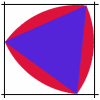#### You may also like### Is There a Theorem?

Draw a square. A second square of the same size slides around the first always maintaining contact and keeping the same orientation. How far does the dot travel?### The Old Goats

A rectangular field has two posts with a ring on top of each post. There are two quarrelsome goats and plenty of ropes which you can tie to their collars. How can you secure them so they can't fight each other but can reach every corner of the field?### Rolling Triangle

The triangle ABC is equilateral. The arc AB has centre C, the arc BC has centre A and the arc CA has centre B. Explain how and why this shape can roll along between two parallel tracks.

# Rolling Around

##### Age 11 to 14Challenge Level

Faisal from Arnold House School offered a strategy for working on this problem:

What we did was take a dart board and rolled it around 4 metre rulers.
It travelled a straight line along the ruler. The bullseye of the board stayed the same distance away from the ruler.
After each corner the bullseye made a curve a quarter of a circle (which is 90 degrees) so after the experiment is over the bullseye would have turned a whole circle.
The distance travelled is the length of the 4 metre rulers plus the circumference of the circle.

We had several more good solutions for the first part of this problem from pupils at Highcliffe Primary School. Whitney and Joe said:

The new shape (i.e. the locus of the centre of the circle) will be a square with rounded corners.

Sam and John explained that the length of the locus ...

... is the perimeter of the old square plus that of the circle.

Oliver from Olchfa School sent us this clearly argued solution with useful diagrams which went beyond squares and generalised for circles rolling round any convex polygons.

Bill from Dana Middle School also gave us a detailed description of the path of the centre of the circle:

As the circle rolls along the outside of the square, its centre traces a path one radius distant from the side of the box, parallel to the side, and the same length as the side, until the circle gets to a corner. At the corner, the point on the circle stays fixed to the corner, while the centre of the circle traces a circular path of radius r around the corner, until the radius (from the corner of the square, now) has swept out a certain angle (in this case, 90 degrees). The sweep begins with the radius at a right angle to the side the circle is leaving, and ends when the radius is at a right angle to the side it is entering. Since it does this four times, the length of the path around the corners of the square equals the circumference of the circle.

In effect, in circumnavigating the corners, the centre of the circle rotates completely around a point on the circle's circumference one time, and, since the distance from the centre to the edge is the same as the distance from the edge to the centre, this part of the path is the length of the circumference. On the straight sections, the length of the path is equal to the length of the sides, making it equal to the perimeter of the square.

The length of the path is the perimeter of the square plus the circumference of the circle.

By like reasoning, when the circle rolls around a triangle, as it rounds the corners the circle will turn around completely once, which results in the centre of the circle tracing out the circumference, which, when added to the perimeter of the triangle will give the length of the path.

In general, as a circle rolls around a convex polygon, the length of the path of the centre of the circle will be the perimeter of the polygon plus the circumference of the circle, the locus of the path being a distance away from the polygon equal to the radius of the circle.

Very thorough explanations; thank you Oliver and Bill .